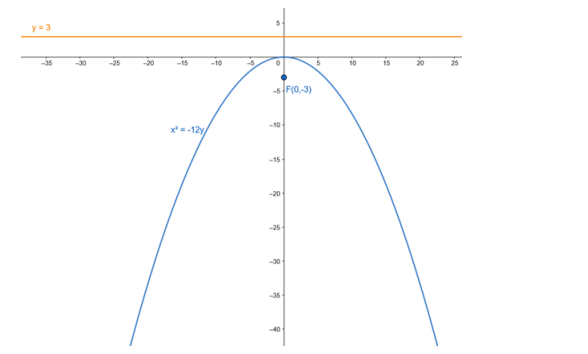# Find the equation of the parabola with focus`
Question:

Find the equation of the parabola with focus $F(0,-3)$ and directrix $y=3$.

Solution:

Given equation of directrix : y = 3

- $y-3=0$

Above equation is of the form, y - a = 0

Focus of the parabola $F(0,-3)$ is of the form $F(0,-a)$

Therefore, a = 3

For directrix with equation $y-a=0$ and focus $(0,-a)$, equation of the parabola is,

$x^{2}=-4 a y$

$\cdot x^{2}=-12 y$